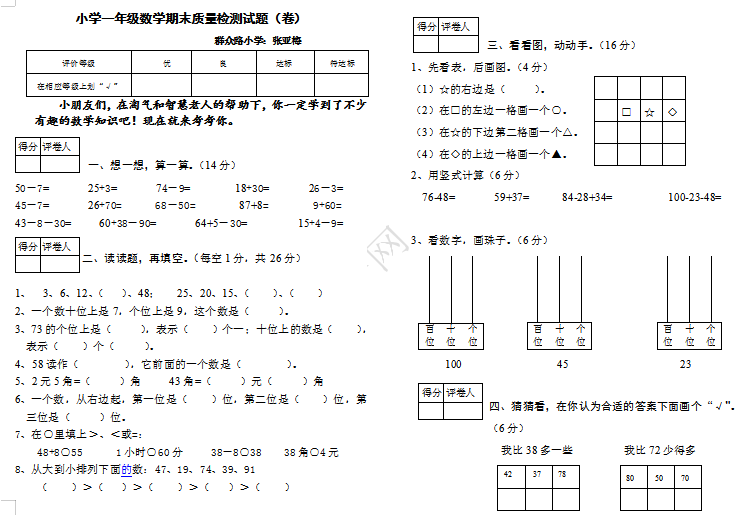评价等级 优 良 达标 待达标 在相应等级上划“√”

50－7=       25+3=       74－9=        18+30=       26－3=

45－7=       26+70=      68－50=        87+8=        9+60=

43－8－30=     60+38－90=       64+5－30=         15+4－9=

1、  3、6、12、（   ）、48；   25、20、15、（    ）、（    ）

2、一个数十位上是7，个位上是9，这个数是（     ）。

3、73的个位上是（     ），表示（    ）个一；十位上的数是（    ），表示（    ）个（     ）。

4、58读作（        ），它前面的一个数是（        ）。

5、2元5角=（     ）角     43角=（     ）元（     ）角

6、一个数，从右边起，第一位是（    ）位，第二位是（    ）位，第三位是（     ）位。